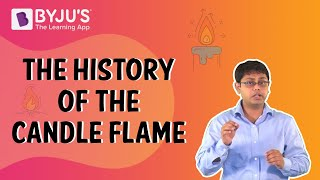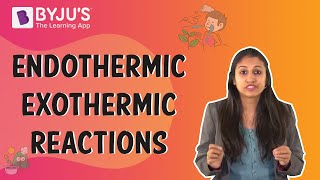Checkout JEE MAINS 2022 Question Paper Analysis : Checkout JEE MAINS 2022 Question Paper Analysis :

# Combustion Reactions Questions

The Combustion Reactions are highly exothermic reactions that take place in between a fuel and an oxidant. The products of this reaction are a mixture of oxidised gaseous products commonly termed as “Smoke”. The reactants require a minimum amount of energy i.e. activation energy to undergo combustion.

 Definition: The reaction in which a fuel burns in the presence of oxygen to form carbon dioxide, water, heat and light is called a Combustion Reaction.

## Combustion Reactions Chemistry Questions with Solutions

Q1: What is the general formula for combustion reaction?

Answer: The general formula of combustion is given as: Fuel + O2

$$\begin{array}{l}\xrightarrow[]{Combustion}\end{array}$$
CO2 + H2O

Q2. Choose the correct option for the statement:

The amount of oxygen during combustion

1. First increases and then decreases
2. Does not vary with time
3. Increases with time
4. Decreases with time

Explanation: The oxygen reacts with the fuel to form carbon dioxide and water. Hence , the oxygen gets used up in the reaction. In the absence of sufficient amounts of oxygen, incomplete combustion takes place.

Q3. Combustion does not necessarily require

1. Flame
2. Oxygen
3. A combustible material
4. Temperature greater than the kindling temperature

Explanation: Some spontaneous combustion materials can ignite themselves at the desired temperatures without the need of a source of flame.

Q4. What is the type of reaction in between alkanes and hydroxyl radicals?

1. Exothermic
2. Endothermic
3. Isochoric
4. Isothermal

Explanation: This is because the O-H bond is much stronger than the C-H bond.

CH3CH3 + OH → CH3CH2 + Ḣ

Q5. Balance the given reaction.

N2 + O2 → N2O5

Answer: The given reaction equation is balanced by the following steps:

1. First, the number of atoms of each of the elements is counted both on the reactants’ and the products’ side.
 Element Reactants’ side Products’ side Nitrogen 2 2 Oxygen 2 5

Hence, we see that the number of nitrogen atoms are equal on both sides. We have to balance the number of oxygen atoms.

1. To balance the oxygen, the molecules containing oxygen are given stoichiometric coefficients such that the product of the coefficient and the initially present atoms of oxygen result in the common multiple of 2 and 5.

N2 + 5O2 → 2N2O5

Now that the oxygen atoms are balanced on both sides. The nitrogen atoms need to be balanced.

2N2 + 5O2 → 2N2O5

Hence, the given equation has been completely balanced.

Q6. How is a chemical change different from a physical change?

Answer: In a chemical change, the new product received has entirely different properties from that of the original reactants. However, in a physical change, no new product is formed.

Q7. “More reactants consumed implies that more products will be formed.” Explain.

Answer: The addition of more reactants would mean that more atoms/ molecules are participating in the reaction. Hence, more reactant molecules would react to form more products.

Q8. Which of the following ions are present in water?

1. H2O+ and OH
2. HO+ and HO
3. H3O+ and OH
4. HO+ and H2O

Explanation: Water undergoes self-ionisation due to which some water molecules dissociates into H+ and OH ions. The H+ ions due to smaller size and very high mobility attacks another water molecule to form H3O+ ions. Hence, H3O+ and OH ions are present in water.

Q9. A hydrocarbon fuel is fully combusted consuming 18.214 g of oxygen to yield 23.118 g of CO2 and 4.729 g of H2O. Find the Empirical formula of the given hydrocarbon.

Answer: As we know, 44 g CO2 contains carbon = 1 mol

23.118 g CO2 contains carbon = (1/44) x 23.118 g = 0.5254 mol

Similarly, 18 g H2O contains hydrogen = 2 mol

4.729 g H2O contains hydrogen = (2/18) x 4.729 = 0.5254 mol

Hence, the given hydrocarbon contains equal moles of carbon and hydrogen. So, the empirical formula of the hydrocarbon must be CH.

Q10. The factor crucial for the beginning of a combustion reaction is/are:

1. Carbon dioxide
2. Water
3. Fuel
4. Fuel, oxygen and heat

Explanation: The combustion reaction requires a fixed temperature in order to undergo combustion. For this purpose, heat, oxygen and fuel are required.

Q11. Do metals form ashes upon combustion?

Answer: The metals react in the presence of oxygen and form metal oxides. This is a combination reaction and since oxygen gets combined with the metal, the mass of the metal increases unlike ashes which are very lighter in mass.

Q12. What must the methane gas be combined with in order to undergo combustion?

Answer: The methane gas must be combined with oxygen to undergo combustion.

Q13. What happens when acetic acid is burnt in the presence of air (excess oxygen)?

Answer: On burning in the presence of air, the acetic acid undergoes a combustion reaction producing carbon dioxide gas and water.

CH3COOH (l) + 2O2 (g)

$$\begin{array}{l}\xrightarrow[]{Combustion}\end{array}$$
2CO2 (g) + 2H2O (g)

Q14. Why do some materials burn with a flame while others burn without a flame?

Answer: The substances that produce a flame when they are burnt undergo an incomplete combustion reaction. Due to the incomplete combustion, carbon is produced as soot which causes the flames. However, the substances that undergo complete combustion do not produce any carbon and hence, do not burn with any flame.

Q15. Why does the wood burn but not iron?

Answer: This is because the wood has less dense structure than that of iron as the structure of wood contains many air pockets. Also, the ignition temperature of wood is lesser than that of carbon. Hence, wood burns easily but not iron. However, iron can be put to combustion by dividing it into very small particles or converting it into iron wool.

## Practise Questions on Carbonyl Compounds

Q1. Can steam cause items to catch fire?

Q2. Acetylene burns in air to form carbon dioxide and water. Give a balanced chemical equation for this reaction and mention the steps involved in balancing.

Q3. Calculate the amount of water produced by the combustion of 16 g methane.

Q4. How many moles of the methane gas are required to produce 22 g of carbon dioxide gas as a result of its combustion?

Q5. Iron is converted into its oxide (Fe3O4) by the action of steam on it. Calculate the mass of iron that will be converted to its oxide by the action of 18 g of steam.

Click the PDF to check the answers for Practice Questions.

## Recommended Videos

#### The History of the Candle Flame#### Endothermic Exothermic Reactions difference